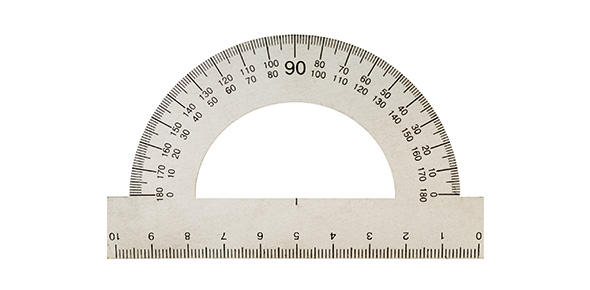# Measurement: Metric And Customary Quiz

15 Questions | Attempts: 507
ShareSettingsConverting metric and customary units to solve problems.

• 1.
To solve the following problem what will you do?2 km  =   __________  m
• A.

Divide 2 by 1000

• B.

Multiply 2 by 100

• C.

Multiply 2 by 1000

• D.

Divide 2 by 100

• 2.
To solve the following problem what will you do?300 seconds =   ____________ minutes
• A.

Divide by 100

• B.

Divide by 60

• C.

Multiply by 60

• D.

Multiply by 100

• 3.
To solve the following problem what will you do?542 centimeters  =   ______________ meters
• A.

Divide by 100

• B.

Multiply by 100

• C.

Divide by 1000

• D.

Multiply by 1000

• 4.
To solve the following problem what will you do?17 feet =   ______________ yards
• A.

Multiply by 12

• B.

Divide by 12

• C.

Divide by 3

• D.

Multiply by 3

• 5.
To solve the following problem what will you do?15 weeks  =   ____________   days
• A.

Multiply by 5

• B.

Multiply by 7

• C.

Divide by 7

• D.

Multiply by 10

• 6.
To solve the following problem what will you do?534.2 millimeters =   ___________ meters
• A.

Multiply by 100

• B.

Divide by 100,000

• C.

Multiply by 1000

• D.

Divide by 1000

• 7.
To solve the following problem what will you do?1460  days =   ____________ years
• A.

Divide by 365

• B.

Divide by 100

• C.

Multiply by 365

• D.

Divide by 12

• 8.
To solve the following problem what will you do?14 miles =  ______________ yards
• A.

Multiply by 5280

• B.

Multiply by 1760

• C.

Divide by 1760

• D.

Multiply by 100

• 9.
To solve the following problem what is the first step?Put in order least to greatest:    1.5 yards             4 feet 3 inches              57 inches
• A.

Change all units into yards

• B.

Change all units into inches

• C.

Change all units into feet

• 10.
To solve the following problem what is the first step? Put in order least to greatest:2832  yards                          7821 feet                     1.2 miles
• A.

Change all units into miles

• B.

• C.

Change them all into yards

• D.

Change them all into feet

• 11.
To solve the following problem what is the first step? Put in order least to greatest: 77 ounces              6 cups                  4.5 pints            2.1 quarts
• A.

Change them all into quarts

• B.

Change them all into cups

• C.

Change them all into ounces

• D.

Change them all into pints

• 12.
When trying to solve measurement problems where you convert from one unit to another the first step is to label which is the larger unit and which is the smaller unit.Next step is to remember the rule:large to small----- multiplysmall to large ------ divideYou multiply or divide by the number of small unit in the large unit.
• A.

True

• B.

False

• 13.
It is important to use the reference sheet given to you when solving measurement problems to check for specific formulas and customary and metric conversions.
• A.

True

• B.

False

• 14.
7.5  tons = _____________ pounds
• A.

7,500

• B.

75,000

• C.

15,000

• D.

14,000

• 15.
3.75 ( or 3  3/4 years) =  _____________ months
• A.

45

• B.

37.5

• C.

42

• D.

36

## Related TopicsBack to top
×

Wait!
Here's an interesting quiz for you.